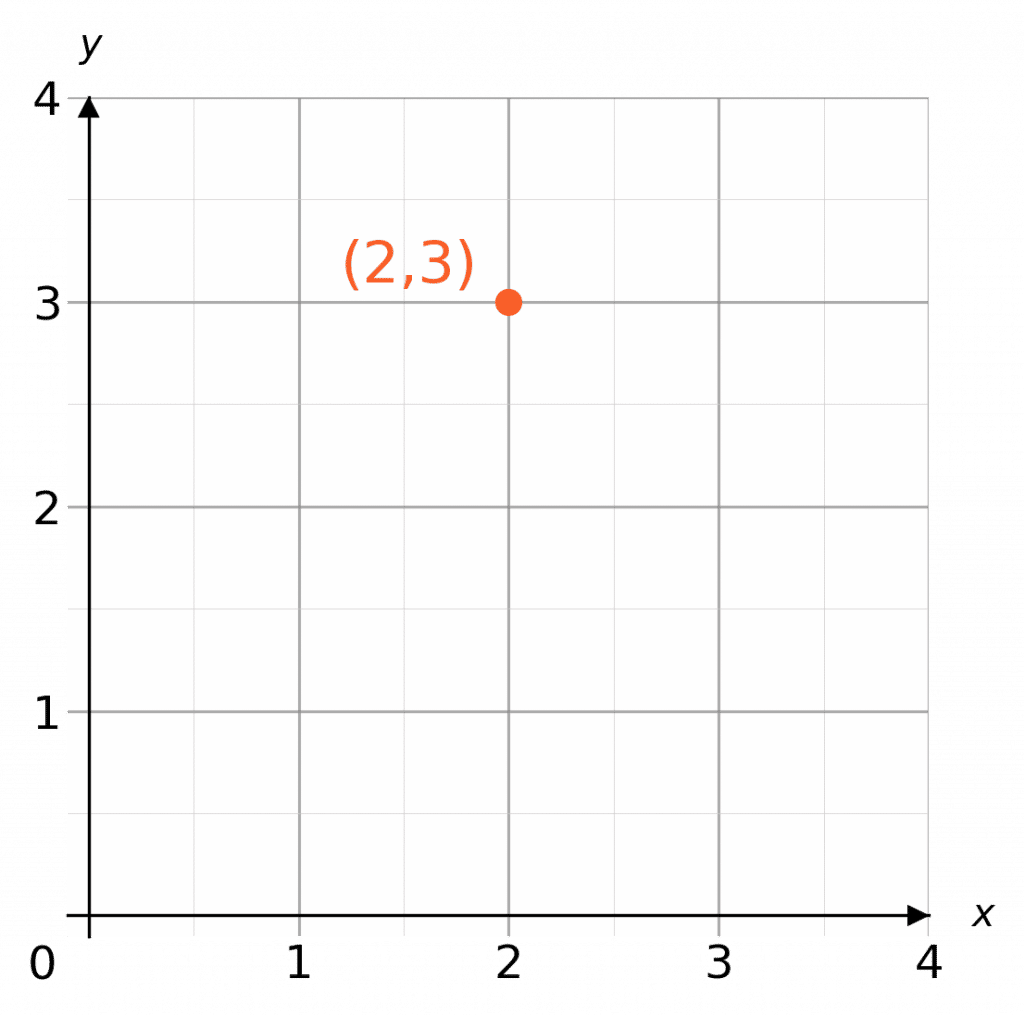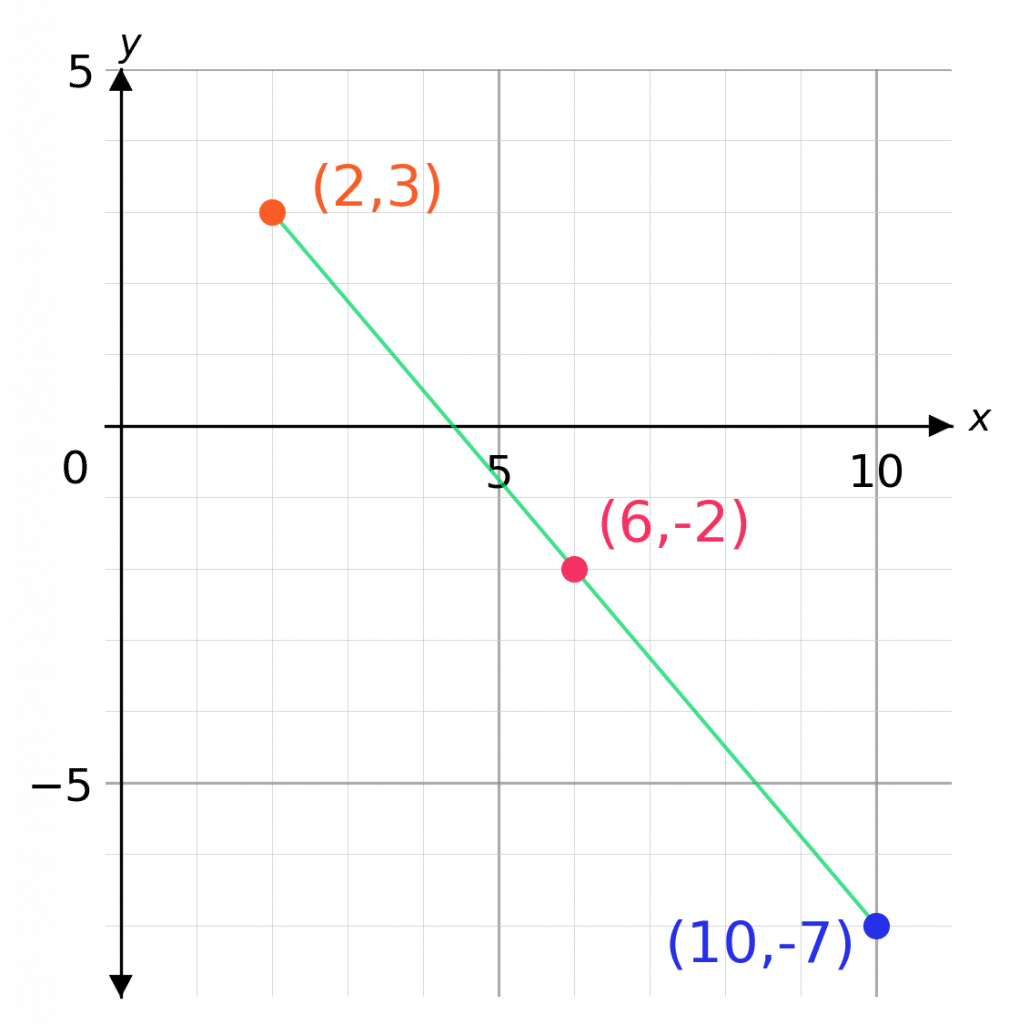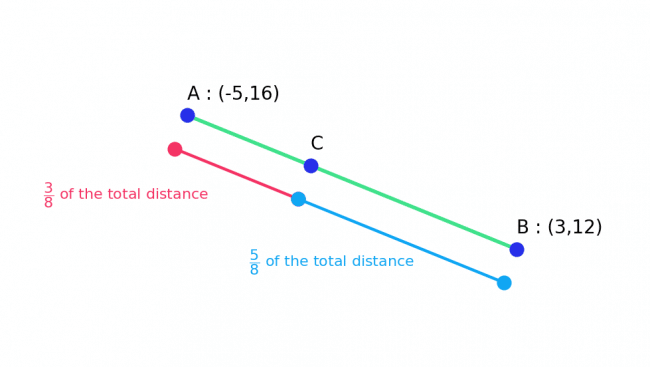# Coordinates and Ratios

GCSEKS3Level 1-3Level 4-5Level 6-7AQAEdexcelEdexcel iGCSEOCRWJEC

## Coordinates and Ratios

Coordinates, denoted by $\textcolor{red}{(x, y)}$, are what we use to communicate where a particular point is located on a pair of coordinate axes.

There are 3 key skills you need to know involving coordinates and ratios for GCSE Maths.

Make sure you are happy with the following topics before continuing:

Level 4-5GCSEKS3AQAEdexcelOCRWJECEdexcel iGCSE

## Skill 1: Plotting Coordinates

Plot the point $(\textcolor{red}{2},\textcolor{blue}{3})$

On the $x$-axes we find $2$, and on the $y$ axes we find $3$ and mark the point with a dot or a cross.

$x = \textcolor{red}{2}$

$y=\textcolor{blue}{3}$Level 1-3GCSEKS3AQAEdexcelOCRWJECEdexcel iGCSE

## Skill 2: Finding the Midpoint of a Line

Find the midpoint of the line segment which joins points

$\textcolor{Orange}{A:(2,3)}$ and $\textcolor{blue}{B:(10,-7)}$

The word midpoint refers to the point which is exactly halfway between the two points in question.

Step 1: Find the half way point of the $x$ coordinates by adding them up and dividing by $2$,

$\text{Midpoint of }x\text{ coordinates } = (2 + 10) \div 2 = 6$

Step 2: Repeat for the $y$ coordinates,

$\text{Midpoint of }y\text{ coordinates} = (-7 + 3) \div 2 = -2$

Step 3: Write the values for the midpoint as a coordinate: $\textcolor{red}{(6, -2)}$

You can also find the midpoint of a line segment, if the two points at either end are drawn on a grid. , just by looking at it.Level 4-5GCSEAQAEdexcelOCRWJECEdexcel iGCSE
Level 6-7GCSEAQAEdexcelOCRWJECEdexcel iGCSE

## Skill 3: Ratios to find Coordinates

Finding a point a certain way between two points is the hardest part of this topic and involves a good knowledge of using ratios.

Example: Points $A$ and $B$ have coordinates $(-5, 16)$ and $(3, 12)$ respectively.

Point $C$ lies on the line segment between points $A$ and $B$ such that $AC:CB = \textcolor{red}{3}:\textcolor{blue}{5}$.

Find the coordinates of point $C$.

Step 1: Find the total parts.

$\textcolor{red}{3}:\textcolor{blue}{5}$ which means there are $\textcolor{limegreen}{8}$ parts in total.

The distance from $A$ to $C$ is $\textcolor{red}{3}$ parts of a total $\textcolor{limegreen}{8}$.Step 2: Find the $x$ value.

Total distance in the $x$ coordinates:

$3 - (-5) = 8$

Next we find $\dfrac{\textcolor{red}{3}}{\textcolor{limegreen}{8}}$of this.

$\dfrac{\textcolor{red}{3}}{\textcolor{green}{8}} \times 8 = 3$

Adding this to the $x$ coordinate of $A$, we get

$x\text{ coordinate of C } = -5 + 3 = -2$

Step 3: Repeat for $y$

Total distance in the $y$ coordinates:

$12 - 16 = -4$

$\dfrac{\textcolor{red}{3}}{\textcolor{green}{8}} \times -4 = -\dfrac{3}{2}$

Adding this to the $y$ coordinate of $A$, we get

$y\text{ coordinate of C } = 16 + \left(-\dfrac{3}{2}\right) = \dfrac{29}{2}$

Therefore, the coordinates of $C$ are $\left(-2, \dfrac{29}{2}\right)$.

You could also write this as $\left(-2, 14.5\right)$.

Level 6-7GCSEAQAEdexcelOCRWJECEdexcel iGCSE

## Coordinates and Ratios Example Questions

$A$ is $- 2$ in the $x$ direction and $2$ in the $y$ direction, so $A = (-2, 2)$.

$B$ is $- 1$ in the $x$ direction and $- 2$ in the $y$ direction, so $B = (-1, -2)$.

$C$ is $3$ in the $x$ direction and $0$ in the $y$ direction, so $C = (3, 0)$.Gold Standard Education

Point $A$ has coordinates $(-2, -2)$.

Point $B$ has coordinates $(0, 3)$.

By taking the average of the $x$ coordinates of $A$ and $B$, the $x$ coordinate of the midpoint is

$\dfrac{-2 + 0}{2} = -1$.

By taking the average of the $y$ coordinates of $A$ and $B$, the $y$ coordinate of the midpoint is

$\dfrac{-2 + 3}{2} = \dfrac{1}{2}$.

Therefore, the coordinates of the midpoint are $\left(-1, \dfrac{1}{2}\right)$.

Note: it is often useful to check the graph to see if your answer looks correct.Gold Standard Education

In a ratio of $2:7$ there are $9$ parts in total, and the distance from $A$ to $C$ constitutes $2$ of those parts. Therefore, the distance from $A$ to $C$ counts for $\dfrac{2}{9}$ of the total distance between $A$ and $B$. So, we’re going to subtract the individual coordinates of $A$ from $B$ to find the distance in both $x$ and $y$, and then we are going to add $\dfrac{2}{9}$ of these respective distances to the coordinates of point $A$.

First, $x$ coordinates: $-16 -(-10) = -6$, then

$\dfrac{2}{9} \times (-6) = -\dfrac{12}{9} = -\dfrac{4}{3}$

Adding this to the $x$ coordinate of $A$, we get

$x\text{ coordinate of C } = -10 + \left(-\dfrac{4}{3}\right) = -\dfrac{34}{3}$

Second, $y$ coordinates: $1 - 37 = -36$, then

$\dfrac{2}{9} \times (-36) = -\dfrac{72}{9} = -8$

Adding this to the $y$ coordinate of $A$, we get

$y\text{ coordinate of C } = 37 + (-8) = 29$

Therefore, the coordinates of $C$ are $\left(-\dfrac{34}{3}, 29\right)$.Gold Standard Education

## Coordinates and Ratios Worksheet and Example Questions

### (NEW) Coordinates & Ratios Exam Style Questions - MME

Level 1-3Level 4-5GCSENewOfficial MME

## Coordinates and Ratios Drill Questions

### Mid points Ratios and Coordinates - Drill Questions

Level 1-3GCSEGold Standard Education

£19.99 /month. Cancel anytime

Level 4-5GCSEKS3

Level 1-3GCSEKS3